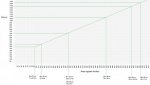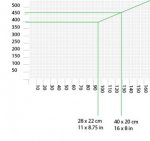# please help by providing an excel equation for a "price per square cm" problem

#### turtleowurtle

##### New member
please help by providing an excel equation for a "price per square cm" problem

Hi everyone,

I'm terrible at maths, so don't know if this is even the right thread for my problem but here goes...

I am a painter. I want the price of my paintings to scale in a linear fashion, but don't want to charge a set amount per square cm. I want the price to decrease as the painting size increases in a straight, linear line.

My cheapest painting is £395 for a 28 x 23cm painting with an area of 644cm2

My most expensive painting is £2950 for a 90 x 90cm painting with an area of 8100cm2

Please tell me the formula I can use in excel to input any value for the cm squared area, that tells me the price for that size.

Thanks!

#### Dr.Peterson

##### Elite Member
I am a painter. I want the price of my paintings to scale in a linear fashion, but don't want to charge a set amount per square cm. I want the price to decrease as the painting size increases in a straight, linear line.

My cheapest painting is £395 for a 28 x 23cm painting with an area of 644cm2

My most expensive painting is £2950 for a 90 x 90cm painting with an area of 8100cm2

Please tell me the formula I can use in excel to input any value for the cm squared area, that tells me the price for that size.
Clearly this is not a problem for a class, so it's ok for me to just tell you the answer, if I can.

We'll have to clarify what you want, but we can figure it out one way or another! And yes, it is basic algebra.

You say you want the price to decrease as size increases; clearly the actual cost of a painting increases, so you must be referring to the price per square centimeter decreasing linearly. Is that what you have in mind?

Alternatively, when you say you want the price to scale linearly, you could mean that you want the cost of a painting to be a linear function of the area, which would be a different thing. I'll assume that is not what you want. It would amount to charging a fixed price per cm2 plus some overhead.

First, the price per square cm of the 644 cm2 painting is 0.613, while for the 8100 cm2 it is 0.364. Using the point-slope formula, this gives the price per cm2 as

P = - 0.0000334(A - 644) + 0.613

so

P = 0.6345 - 0.0000334A

Then the cost is the price times the area, giving

C = (0.6345 - 0.0000334A)A = 0.6345A - 0.0000334A2

As you see, when the price per cm2 increases linearly, the cost increases quadratically. This implies it will rise to a peak cost, after which the cost of larger paintings would actually decrease. In fact, the price per cm2, by my formula for P, actually reaches zero at about 19,000 cm2, and then goes negative. Presumably you'll never make one that large? That's the danger of decreasing prices linearly.

Does this look like what you want?

#### turtleowurtle

##### New member
Clearly this is not a problem for a class, so it's ok for me to just tell you the answer, if I can.

We'll have to clarify what you want, but we can figure it out one way or another! And yes, it is basic algebra.

You say you want the price to decrease as size increases; clearly the actual cost of a painting increases, so you must be referring to the price per square centimeter decreasing linearly. Is that what you have in mind?

Alternatively, when you say you want the price to scale linearly, you could mean that you want the cost of a painting to be a linear function of the area, which would be a different thing. I'll assume that is not what you want. It would amount to charging a fixed price per cm2 plus some overhead.

First, the price per square cm of the 644 cm2 painting is 0.613, while for the 8100 cm2 it is 0.364. Using the point-slope formula, this gives the price per cm2 as

P = - 0.0000334(A - 644) + 0.613

so

P = 0.6345 - 0.0000334A

Then the cost is the price times the area, giving

C = (0.6345 - 0.0000334A)A = 0.6345A - 0.0000334A2

As you see, when the price per cm2 increases linearly, the cost increases quadratically. This implies it will rise to a peak cost, after which the cost of larger paintings would actually decrease. In fact, the price per cm2, by my formula for P, actually reaches zero at about 19,000 cm2, and then goes negative. Presumably you'll never make one that large? That's the danger of decreasing prices linearly.

Does this look like what you want?

Thanks so much for helping.

I have attached a screen grab of the method I currently use for calculating price. As you can see, price always goes up, but at a slower rate as the line is not 45 degrees. I'm sorry if I didn't explain it properly in my initial post. I also may have used the word linear in the wrong context. I'm really really hopeless at math, so forgive me.

Does this screen shot makes sense of what I'm after? I just want to be able to type in a height and width value into a cell in a spread sheet, and the forumla tell me the price, using my scaling method from the screengrab. I cant see the price ever going down at any point, regardless of size :/ bit confused about that part of your answer.#### Dr.Peterson

##### Elite Member
Thanks so much for helping.

I have attached a screen grab of the method I currently use for calculating price. As you can see, price always goes up, but at a slower rate as the line is not 45 degrees. I'm sorry if I didn't explain it properly in my initial post. I also may have used the word linear in the wrong context. I'm really really hopeless at math, so forgive me.

Does this screen shot makes sense of what I'm after? I just want to be able to type in a height and width value into a cell in a spread sheet, and the forumla tell me the price, using my scaling method from the screengrab. I cant see the price ever going down at any point, regardless of size :/ bit confused about that part of your answer.

View attachment 9320View attachment 9321
It appears that the part in your question about price decreasing was some sort of a misstatement, and the alternative I suggested was in fact what you want:
Alternatively, when you say you want the price to scale linearly, you could mean that you want the cost of a painting to be a linear function of the area, which would be a different thing. I'll assume that is not what you want. It would amount to charging a fixed price per cm2 plus some overhead.
Taking it this way, we want a linear equation relating price (not price per cm2) to area, passing through the points (644, 395) and (8100, 2950). The slope is (2950-395)/(8100-644) = 0.342677, so the equation is P = 0.342677(A - 644) + 395 = 0.342677A + 174.32.

As a check, for A = 395, this gives 0.342677(644) + 174.32 = 395, for A = 8100, it gives 0.342677(8100) + 174.32 = 2950. Taking a number from your graph, for A = 800, I get a price of 0.342677(800) + 174.32 = 448.46, which looks right.

What this formula says, in effect, is that you are charging £0.34 per square centimeter, plus £174.32 for overhead, though you don't have to think of it that way.

Does this look good to you? It certainly makes more sense than my original interpretation.

#### turtleowurtle

##### New member
It appears that the part in your question about price decreasing was some sort of a misstatement, and the alternative I suggested was in fact what you want:

Taking it this way, we want a linear equation relating price (not price per cm2) to area, passing through the points (644, 395) and (8100, 2950). The slope is (2950-395)/(8100-644) = 0.342677, so the equation is P = 0.342677(A - 644) + 395 = 0.342677A + 174.32.

As a check, for A = 395, this gives 0.342677(644) + 174.32 = 395, for A = 8100, it gives 0.342677(8100) + 174.32 = 2950. Taking a number from your graph, for A = 800, I get a price of 0.342677(800) + 174.32 = 448.46, which looks right.

What this formula says, in effect, is that you are charging £0.34 per square centimeter, plus £174.32 for overhead, though you don't have to think of it that way.

Does this look good to you? It certainly makes more sense than my original interpretation.

That's great, thanks so much for your help. Really appreciate it.